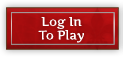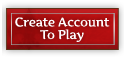Forum Home > News & Announcements > AnnouncementsNew PostsFAQSearchRegisterLogin

#Mild Release: Patch sizes, Animal parts

 Post ReplyPage  <123>
AuthorTopic SearchTopic Options
geofreyPostmaster GeneralJoined: 31 May 2011
Status: Offline
Points: 1013Posted: 02 Aug 2012 at 20:41Quackers wrote:geofrey wrote:Can you list a recipe page on the site/forum? While I understand light grey skin and dark grey skin are different, I don't know what they are called and it is hard to tell them apart when not right next to each other. If no full recipe page, maybe allow a mouse over on research to list the name of the ingredient?

Still a work in progress: http://elgea.illyriad.co.uk/#/Player/Profile/97413

your my heroSalarariusPostmasterJoined: 26 Sep 2011
Location: USA
Status: Offline
Points: 519Posted: 02 Aug 2012 at 21:27
Harvesting caravan's do not show up as harvesting in the "Trade Overview" screen.SalarariusPostmasterJoined: 26 Sep 2011
Location: USA
Status: Offline
Points: 519Posted: 02 Aug 2012 at 22:29Quackers wrote:geofrey wrote:Can you list a recipe page on the site/forum? While I understand light grey skin and dark grey skin are different, I don't know what they are called and it is hard to tell them apart when not right next to each other. If no full recipe page, maybe allow a mouse over on research to list the name of the ingredient?

Still a work in progress: http://elgea.illyriad.co.uk/#/Player/Profile/97413

How did you get that in your profile?  When I try to paste the HTML (slightly modified) in:

<div><span>Sword</span><span class="name base swords_24" data="c=3" title="Sword"></span></div>
<div><span>Bow</span><span class="name base bows_24" data="c=4" title="Bow"></span></div>
<div><span>Spear</span><span class="name base spears_24" data="c=5" title="Spear"></span></div>

it shows up in the edit window but then disappears when I press Update Profile.  I'd like to see that list with the words in front so I don't have to hover over every icon to see what it is.  I find it easier to remember the names and than the icons.

ThanksQuackersForum WarriorJoined: 19 Nov 2011
Location: Jeff City
Status: Offline
Points: 435Posted: 03 Aug 2012 at 01:06
Updated thanks to Tallica.

(copy this and past on Your Profile in game)

Basic Resources
[@c=183] [@c=295]  [@c=296] [@c=17] [@c=19] [@c=21] [@c=23] [@c=25]

Advanced Resources
[@c=1] [@c=2] [@c=3] [@c=4] [@c=5] [@c=6] [@c=7] [@c=8] [@c=9] [@c=10] [@c=11] [@c=12] [@c=420]

Crafted Horses
[@c=33] [@c=34] [@c=35] [@c=36] [@c=37]

Crafted Swords
[@c=40] [@c=41] [@c=42] [@c=43] [@c=44] [@c=46] [@c=48] [@c=49] [@c=50] [@c=51] [@c=52] [@c=53] [@c=54][@c=57] [@c=58] [@c=59] [@c=60] [@c=61] [@c=62] [@c=63] [@c=64]

Crafted Bows
[@c=67][@c=68][@c=69][@c=70][@c=72][@c=73][@c=74][@c=75][@c=76][@c=77][@c=78][@c=79][@c=80][@c=81][@c=82][@c=83][@c=84][@c=85][@c=86][@c=87][@c=88][@c=89]

Crafted Spears
[@c=91] [@c=92] [@c=93] [@c=94] [@c=95] [@c=96] [@c=97] [@c=98] [@c=99] [@c=100] [@c=101] [@c=102] [@c=103] [@c=104] [@c=105] [@c=106] [@c=107] [@c=108] [@c=109]  [@c=110] [@c=111] [@c=112] [@c=113] [@c=114] [@c=115] [@c=116]

Crafted Armor
[@c=120] [@c=121] [@c=122] [@c=123] [@c=124] [@c=125] [@c=126] [@c=127] [@c=128] [@c=129] [@c=130] [@c=131] [@c=132] [@c=133] [@c=134] [@c=135] [@c=136] [@c=137] [@c=138] [@c=139]
[@c=141] [@c=142] [@c=143] [@c=144] [@c=145] [@c=146] [@c=147] [@c=148] [@c=149] [@c=150] [@c=151] [@c=153] [@c=154] [@c=155] [@c=156] [@c=157] [@c=158] [@c=159]
[@c=160] [@c=161] [@c=162] [@c=163] [@c=165] [@c=166] [@c=167] [@c=168] [@c=169] [@c=170] [@c=171] [@c=172] [@c=173] [@c=174] [@c=175] [@c=176] [@c=177] [@c=178] [@c=179]

Minerals
[@c=417] [@c=193] [@c=194] [@c=195] [@c=196] [@c=197][@c=198] [@c=199] [@c=200][@c=201] [@c=202] [@c=203] [@c=204] [@c=205] [@c=206] [@c=207][@c=208] [@c=209] [@c=210][@c=211] [@c=212] [@c=213]

Herbs
[@c=416] [@c=224] [@c=225] [@c=226] [@c=227] [@c=228] [@c=229] [@c=230] [@c=231] [@c=232] [@c=233] [@c=234] [@c=235] [@c=236] [@c=237] [@c=238] [@c=239] [@c=240] [@c=241] [@c=242] [@c=243] [@c=244] [@c=245] [@c=246] [@c=247] [@c=248] [@c=249] [@c=250] [@c=251] [@c=252] [@c=253] [@c=254]

Animal Parts
[@c=186] [@c=298] [@c=299] [@c=300][@c=301] [@c=302] [@c=303] [@c=304] [@c=305] [@c=306] [@c=307][@c=308] [@c=309] [@c=310][@c=311] [@c=312] [@c=313] [@c=314] [@c=315] [@c=316] [@c=317][@c=318] [@c=319] [@c=320][@c=321] [@c=322] [@c=323] [@c=324] [@c=325] [@c=326] [@c=327][@c=328] [@c=329] [@c=330][@c=331] [@c=332] [@c=333] [@c=334] [@c=335] [@c=336] [@c=337][@c=338] [@c=339] [@c=340][@c=341] [@c=342] [@c=343] [@c=344] [@c=345] [@c=346] [@c=347][@c=348] [@c=349] [@c=350][@c=351] [@c=352] [@c=353] [@c=354] [@c=355] [@c=356] [@c=357][@c=358] [@c=359] [@c=360][@c=361] [@c=362] [@c=363] [@c=364] [@c=365] [@c=366] [@c=367][@c=368] [@c=369] [@c=370][@c=371] [@c=372] [@c=373] [@c=374] [@c=375] [@c=376] [@c=377][@c=378] [@c=379] [@c=380][@c=381] [@c=382] [@c=383] [@c=384] [@c=385] [@c=386] [@c=387][@c=388] [@c=389] [@c=390][@c=391] [@c=392] [@c=393] [@c=394] [@c=395] [@c=396] [@c=397][@c=398] [@c=399] [@c=400][@c=401] [@c=402] [@c=403] [@c=404] [@c=405] [@c=406] [@c=407][@c=408] [@c=409] [@c=410] [@c=411] [@c=412] [@c=413] [@c=414] [@c=415]

Exotic/Elemental
[@c=255] [@c=256] [@c=257] [@c=258] [@c=290] [@c=291]  [@c=292] [@c=293]

Discoverie
Elf: [@c=422] [@c=47] [@c=71]
Human: [@c=423] [@c=65] [@c=152]
Dwarf: [@c=424 ][@c=45]  [@c=164]
Orc: [@c=425] [@c=55] [@c=56]

Blank Code
[@c=13] [@c=14] [@c=15] [@c=16] [@c=18] [@c=20] [@c=22] [@c=24] [@c=26] [@c=27] [@c=28] [@c=29] [@c=30] [@c=31] [@c=32] [@c=38] [@c=39] [@c=66] [@c=117] [@c=118] [@c=119] [@c=140] [@c=180] [@c=181] [@c=182] [@c=184] [@c=185] [@c=187] [@c=188] [@c=189] [@c=190] [@c=191] [@c=192] [@c=214] [@c=215] [@c=216] [@c=217] [@c=218] [@c=219] [@c=220] [@c=221] [@c=222] [@c=223] [@c=259] [@c=260][@c=261] [@c=262] [@c=263] [@c=264] [@c=265] [@c=266] [@c=267] [@c=268] [@c=269] [@c=270] [@c=271] [@c=272] [@c=273] [@c=274] [@c=275] [@c=276] [@c=277] [@c=278] [@c=279] [@c=280] [@c=281] [@c=282] [@c=283] [@c=284] [@c=285] [@c=286] [@c=287] [@c=288] [@c=289] [@c=294] [@c=297] [@c=418] [@c=419] [@c=421]

Edited by Quackers - 19 Aug 2012 at 21:05
Make it your ambition to lead a quiet life, to mind your own business and to work with your hands, so that your daily life may win the respect of outsiders and so you will not be dependent on anybody.MakanalaniGreenhornJoined: 20 Nov 2010
Status: Offline
Points: 67Posted: 03 Aug 2012 at 01:38
Where do you find your skinners because my skinner guild and marketplace do not have them?

Edited by Makanalani - 03 Aug 2012 at 01:38
"Life is a beautiful struggle"

-New IGN: Mak (Dark Blight)QuackersForum WarriorJoined: 19 Nov 2011
Location: Jeff City
Status: Offline
Points: 435Posted: 03 Aug 2012 at 02:03Makanalani wrote: Where do you find your skinners because my skinner guild and marketplace do not have them?

Skinners show up in marketplace after you research butchery
Make it your ambition to lead a quiet life, to mind your own business and to work with your hands, so that your daily life may win the respect of outsiders and so you will not be dependent on anybody.SalarariusPostmasterJoined: 26 Sep 2011
Location: USA
Status: Offline
Points: 519Posted: 03 Aug 2012 at 02:08
Thanks so much, here is what I was looking for but what you posted had what I didn't know in it.

Thanks!

Edited by Salararius - 20 Aug 2012 at 00:11QuackersForum WarriorJoined: 19 Nov 2011
Location: Jeff City
Status: Offline
Points: 435Posted: 03 Aug 2012 at 02:16Salararius wrote:Thanks so much, here is what I was looking for but what you posted had what I didn't know in it.Thanks!

Yea I wanted to give you the code I typed but then I noticed all the blank code that did nothing. Didn't want you to have to shift through and figure all that stuff out as well. :P

Your profile looks great, really nice job with the labeling. Oh and here is your profile if people want to see: http://elgea.illyriad.co.uk/#/Player/Profile/66699
Make it your ambition to lead a quiet life, to mind your own business and to work with your hands, so that your daily life may win the respect of outsiders and so you will not be dependent on anybody.lokifeysonForum WarriorJoined: 29 Jul 2010
Status: Offline
Points: 211Posted: 03 Aug 2012 at 06:50
dude yes, just made me like the update more :)

i was saying we needed exactly this :)tallicaForum WarriorJoined: 27 Jun 2011
Location: Seattle, WA
Status: Offline
Points: 378Posted: 18 Aug 2012 at 21:13
Quackers missed a couple of crafted weapons (and maybe armors?) which I've included in this list: (you can copy this to wherever you'd like)

Basic Resources
[@c=183][@c=17][@c=19][@c=21][@c=23][@c=25][@c=295][@c=296]

Advanced Resources
[@c=1][@c=2][@c=12][@c=7][@c=5][@c=3][@c=4][@c=6][@c=8][@c=9][@c=10][@c=11][@c=420]

Minerals
[@c=417][@c=193][@c=194][@c=195][@c=196][@c=197][@c=198][@c=199][@c=200][@c=201][@c=202][@c=203][@c=204][@c=205][@c=206][@c=207][@c=208][@c=209][@c=210][@c=211][@c=212]

Herbs
[@c=416][@c=253][@c=224][@c=225][@c=226][@c=227][@c=228][@c=229][@c=230][@c=231][@c=232][@c=233][@c=234][@c=235][@c=236][@c=237][@c=238][@c=239][@c=240][@c=241][@c=242][@c=243][@c=244][@c=245][@c=246][@c=247][@c=248][@c=249][@c=250][@c=251]
[@c=252]

Animal Parts
[@c=186][@c=298][@c=299][@c=300][@c=301][@c=302][@c=303][@c=304][@c=305][@c=306][@c=307][@c=308][@c=309][@c=310][@c=311][@c=312][@c=313][@c=314][@c=315][@c=316][@c=317][@c=318][@c=319][@c=320][@c=321][@c=322][@c=323][@c=324][@c=325][@c=326][@c=327][@c=328][@c=329][@c=330][@c=331][@c=332][@c=333][@c=334][@c=335][@c=336][@c=337][@c=338][@c=339][@c=340][@c=341][@c=342][@c=343][@c=344][@c=345][@c=346][@c=347][@c=348][@c=349][@c=350][@c=351][@c=352][@c=353][@c=354][@c=355][@c=356][@c=357][@c=358][@c=359][@c=360][@c=361][@c=362][@c=363][@c=364][@c=365][@c=366][@c=367][@c=368][@c=369][@c=370][@c=371][@c=372][@c=373][@c=374][@c=375][@c=376][@c=377][@c=378][@c=379][@c=380][@c=381][@c=382][@c=383][@c=384][@c=385][@c=386][@c=387][@c=388][@c=389][@c=390][@c=391][@c=392][@c=393][@c=394][@c=395][@c=396][@c=397][@c=398][@c=399][@c=400][@c=401][@c=402][@c=403][@c=404][@c=405][@c=406][@c=407][@c=408][@c=409][@c=410][@c=411][@c=412][@c=413][@c=414][@c=415]

[@c=290][@c=291][@c=292][@c=293]

[@c=255][@c=256][@c=257][@c=258]

Swords
[@c=40][@c=41][@c=42][@c=43][@c=44][@c=45][@c=46][@c=47][@c=48][@c=50][@c=51][@c=52][@c=53][@c=54][@c=55][@c=56][@c=57][@c=58][@c=59][@c=60][@c=61][@c=62][@c=63][@c=64][@c=65][@c=49]

Bows
[@c=67][@c=68][@c=69][@c=70][@c=71][@c=72][@c=73][@c=74][@c=75][@c=76][@c=77][@c=78][@c=79][@c=80][@c=81][@c=82][@c=83][@c=84][@c=85][@c=86][@c=87][@c=88][@c=89]

Spears
[@c=91][@c=92][@c=93][@c=94][@c=95][@c=96][@c=97][@c=98][@c=99][@c=100][@c=101][@c=102][@c=103][@c=104][@c=105][@c=106][@c=107][@c=108][@c=109][@c=110][@c=111][@c=112][@c=113][@c=114][@c=115][@c=116]

Horses
[@c=33][@c=34][@c=35][@c=36][@c=37]

[@c=422][@c=423][@c=424][@c=425]

Leather Armors
[@c=120][@c=121][@c=122][@c=123][@c=124][@c=125][@c=126][@c=127][@c=128][@c=129][@c=130][@c=131][@c=132][@c=133][@c=134][@c=135][@c=136][@c=137][@c=138][@c=139]

Chain Armors
[@c=141][@c=142][@c=143][@c=144][@c=145][@c=146][@c=147][@c=148][@c=149][@c=150][@c=151][@c=152][@c=153][@c=154][@c=155][@c=156][@c=157][@c=158][@c=159]

Plate Armors
[@c=161][@c=162][@c=163][@c=164][@c=165][@c=166][@c=167][@c=168][@c=169][@c=170][@c=171][@c=172][@c=173][@c=174][@c=175][@c=176][@c=177][@c=178][@c=179]

Edited by tallica - 18 Aug 2012 at 21:15Post ReplyPage  <123>Tweet

 Forum Jump -- Select Forum --  News  Announcements   - Contests!  Rules  Help  General Questions  Strategies, Tips & Tricks  Technical Support  Elgea   - Alliance Recruitment   - Politics & Diplomacy   - Trade Chat  Broken Lands   - Alliance Recruitment   - Politics & Diplomacy   - Trade Chat  Events   - Tournament I - Hold the Flags!   - Tournament II - Bloodthirst For Knowledge   - Tournament III - Harness the Elementals   - Tournament IV - The Undead Horde   - Tournament V - 2nd Anniversary   - Tournament VI - Dark Harvest   - Tournament VII - Champions Return   - Tournament VIII - The Ruby Jubilee   - Tournament IX - The Tinkers' Tournament   - Tournament X - The Chill of Winter  Lore   - From the Herald  The Caravanserai  The Travellers' Tale   - Fiction   - Histories   - Art  Suggestions & Game Enhancements   - Implemented  Fansites  Technology & data  UIv2  General Discussion   - Side Quests! Forum PermissionsYou cannot post new topics in this forumYou cannot reply to topics in this forumYou cannot delete your posts in this forumYou cannot edit your posts in this forumYou cannot create polls in this forumYou cannot vote in polls in this forum

Forum Software by Web Wiz Forums® version 11.10
Copyright ©2001-2017 Web Wiz Ltd.